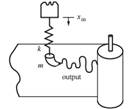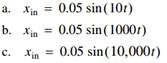Create an Account

Home / Questions / A cheap mechanically actuated strip chart recording system consisting of a plotter head of...

A cheap mechanically actuated strip chart recording system consisting of a plotter head of mass m driven by an elastic belt of stiffness k is illustrated in the following figure The input

A cheap, mechanically actuated, strip chart recording system consisting of a plotter head of mass m driven by an elastic belt of stiffness k is illustrated in the following figure.The input displacement xin is coupled mechanically to the pen head through the spring. The spring constant k is 32.4 N/m and the mass of the pen head is 0.10 kg. Derive the differential equation of motion for the pen head, given the displacement input. Assume that the damping constant between the pen and paper is 10 Ns/m. Using the procedure in Section 4.10.2, determine the steady state output displacement magnitude and phase angle for each steady state input that follows. Explain the differences between the output and input displacements and phase angles.Jul 27 2020 View more View LessSubscribe To Get Solution